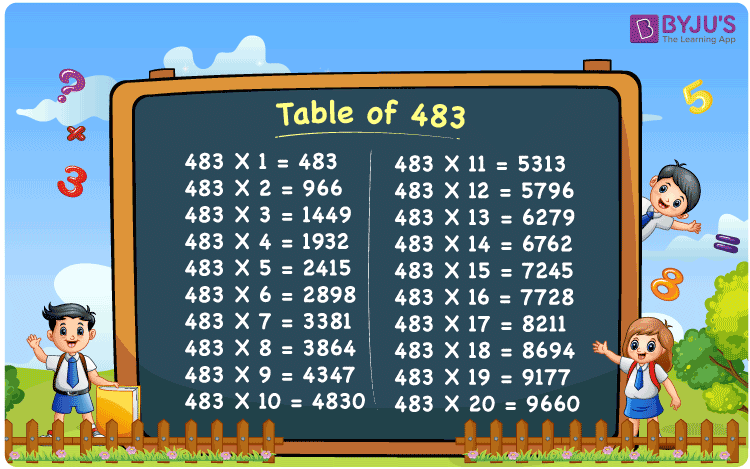Checkout JEE MAINS 2022 Question Paper Analysis : Checkout JEE MAINS 2022 Question Paper Analysis :

# Table of 483

The table of 483 is the multiplication table obtained from the multiplication of 483 by consecutive natural numbers. We know that maths tables are helpful for students preparing for competitive exams. These tables will help them to solve the problems quickly and more accurately. In this article, you will learn one of the maths tables called the table of 483 with the help of a chart and example. Also, download the PDF of the 483 times table from the below-given link.

## Table of 483 Chart

The below chart is used to memorise the table of 483 quickly.## What is the 483 Times Table?

The 483 times table is the repeated addition of 483 to get the multiples of 483 as shown below. For example, we can get the value of 483 times 2 as 483 × 2 = 483 + 483 = 966.

 Using multiplication Using repeated addition 483 × 1 = 483 483 483 × 2 = 966 483 + 483 = 966 483 × 3 = 1449 483 + 483 + 483 = 1449 483 × 4 = 1932 483 + 483 + 483 + 483 = 1932 483 × 5 = 2415 483 + 483 + 483 + 483 + 483 = 2415 483 × 6 = 2898 483 + 483 + 483 + 483 + 483 + 483 = 2898 483 × 7 = 3381 483 + 483 + 483 + 483 + 483 + 483 + 483 = 3381 483 × 8 = 3864 483 + 483 + 483 + 483 + 483 + 483 + 483 + 483 = 3864 483 × 9 = 4347 483 + 483 + 483 + 483 + 483 + 483 + 483 + 483 + 483 = 4347 483 × 10 = 4830 483 + 483 + 483 + 483 + 483 + 483 + 483 + 483 + 483 + 483 = 4830

## Multiplication Table of 483

Students can learn and memorise the multiplication table of 483 up to 20 times.

 483 × 1 = 483 483 × 2 = 966 483 × 3 = 1449 483 × 4 = 1932 483 × 5 = 2415 483 × 6 = 2898 483 × 7 = 3381 483 × 8 = 3864 483 × 9 = 4347 483 × 10 = 4830 483 × 11 = 5313 483 × 12 = 5796 483 × 13 = 6279 483 × 14 = 6762 483 × 15 = 7245 483 × 16 = 7728 483 × 17 = 8211 483 × 18 = 8694 483 × 19 = 9177 483 × 20 = 9660

## Solved Example

Question:

What is the value of 483 times 13?

Solution:

483 times 13

= 483 × 13

Using the table of 483, 483 × 13 = 6279.

= 6279
Therefore, the value of 483 times 13 is 6279.

## Frequently Asked Questions on Table of 483

### How do you write the multiplication table for 483?

We can write the multiplication table for 483 by multiplying 483 by natural numbers as given below:
483 × 1 = 483
483 × 2 = 966
483 × 3 = 1449
483 × 4 = 1932
483 × 5 = 2415

### What is the result when 483 is multiplied by 9?

When 483 is multiplied by 9, we get 4347, i.e. 483 × 9 = 4347.

### Is 483 in the 3 times table?

Yes, 483 comes in the 3 times table when 3 is multiplied by 161, which means 3 × 161 = 483.